## Test Results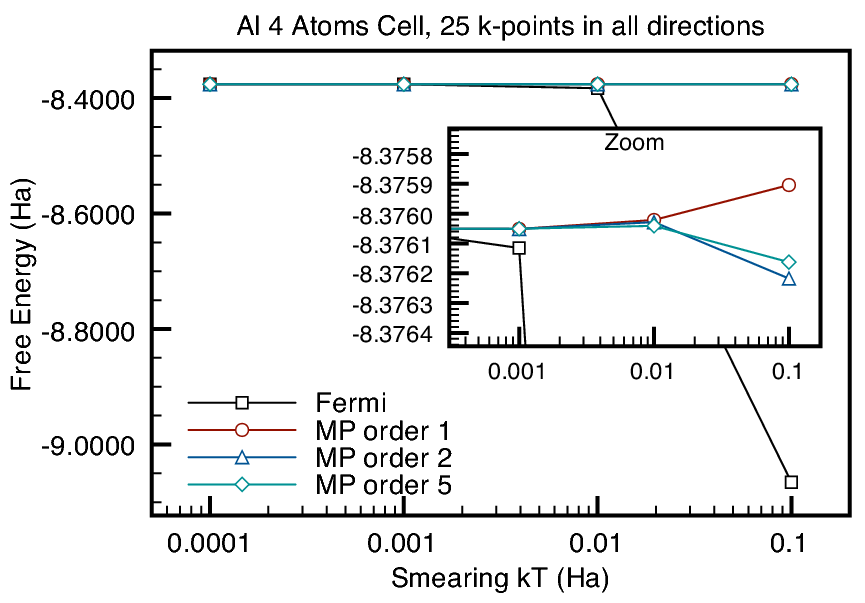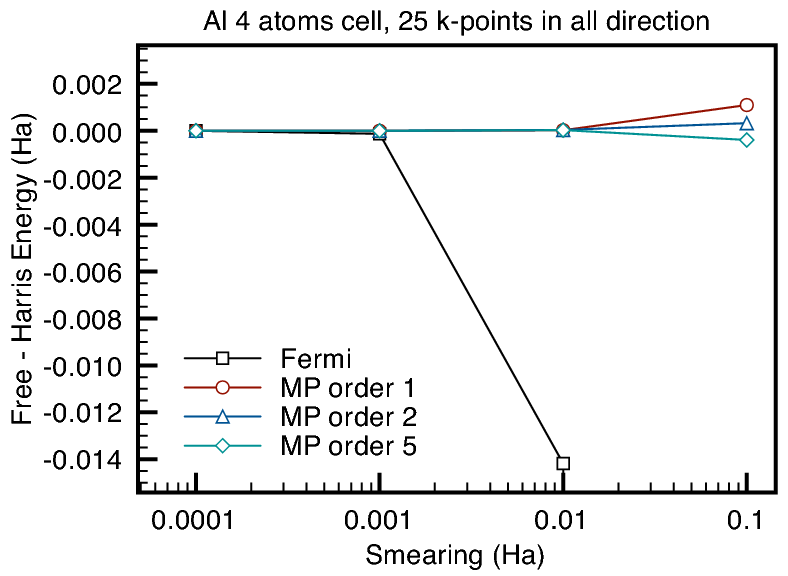Figure 6 shows the comparison between calculations on a 4 atom unit cell aluminium bulk using Fermi and Methfessel-Paxton smearing methods. We only did non-self-consistency calculations. We can see that Fermi smearing leads to different energy results as we increase smearing factor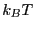. We can see that the difference between Free energy and the calculated ground-state energy (we called it Harris energy) also increased dramatically for Fermi smearing. This is expected as the smearing does correspond to the physical temperature and asincreases we depart from the zero-temperature regime. On the other hand, the smearing in Methfessel-Paxton method is just a parameter used in the approximation. And we can see that increasinghas small effect on the energies calculated using Methfessel-Paxton smearing and results also improve as the order of Hermite polynomials used increases. This means a relatively large smearing factor can be used under Methfessel-Paxton approximation, which allows fewer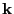-points. To be more specific, figure 7 shows that for smearing factor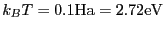, (corresponding to smearing temperature of about 31565.51 K), we reach-point convergence at about 10 Bloch space () points per each reciprocal lattice direction, while the total energy will be withinHa of the ground state energies calculated using low smearing and large number of-points. This is compared with the requirement of 25-points for convergence with a small smearing factor, see figure 8.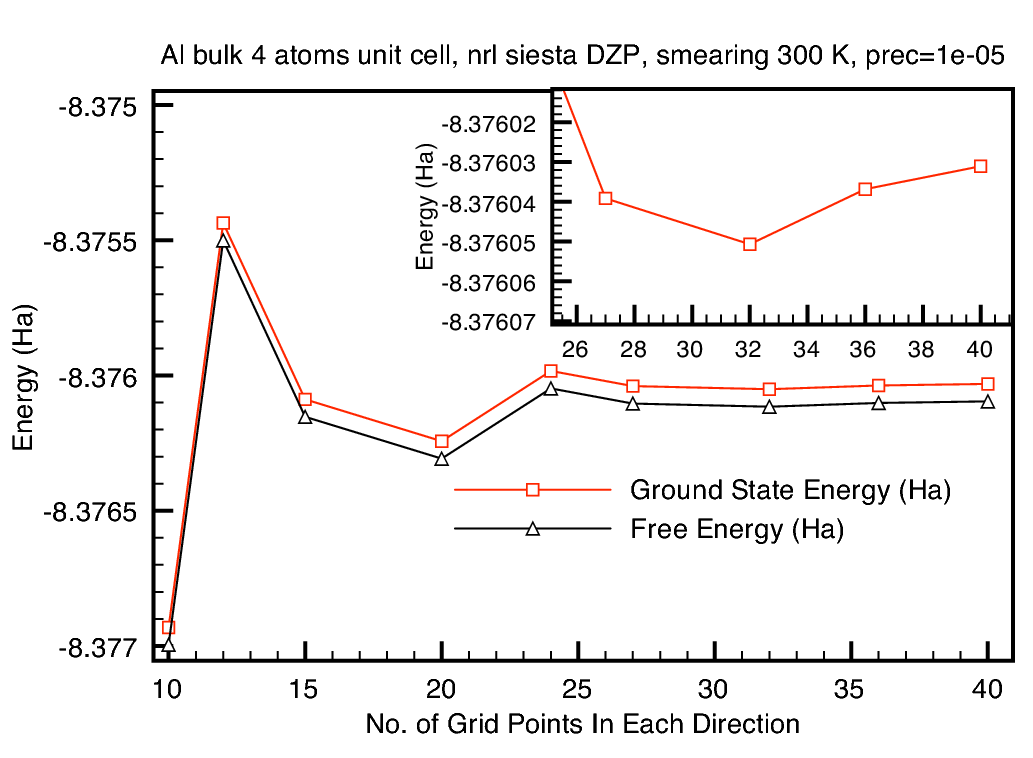Lianheng Tong 2011-03-02